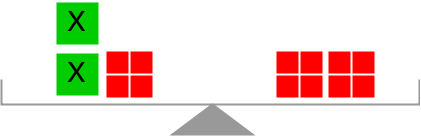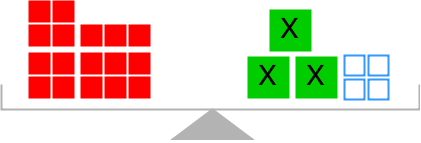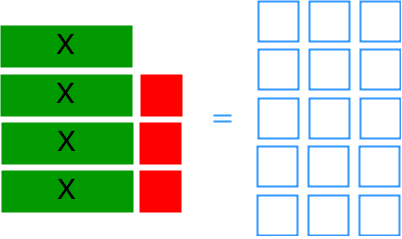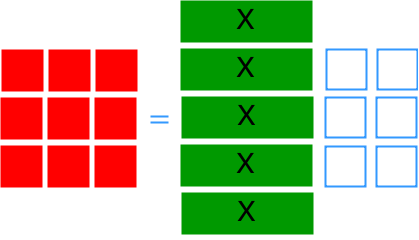# Solving two-step linear equations using addition and subtraction: $ax + b = c$

### Solving two-step linear equations using addition and subtraction: $ax + b = c$

In this section, we will look at linear equation questions which involve two kinds of operations: addition and subtraction. We will deal with two-step linear equations modeled by diagrams as a start. Then, we will learn how to solve algebraic equations and related word problems.

#### Lessons

• 1.
Find x in the equation modelled by each diagram.
a)b)• 2.
Find x in the equation modelled by algebra tiles.
a)b)• 3.
Solve.
a)
$7x + 9 = 21$

b)
$98 - 2x = 14$

c)
$- 35 = 6x + 49$

d)
$- 4x - 11 = 109$

• 4.
The total admission fee of an aquarium for 30 students is $240 after a group discount of$3 for each person. What is the original admission fee before the discount?